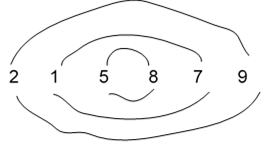# Circle Sort in C++

C++Server Side ProgrammingProgramming

Circle Sort is an interesting sorting algorithm to sort a given array of elements. The algorithm compares the elements of the array diametrically and once the elements in one part is sorted, then continuously sort the other end of the array diametrically.

Example

Let us visualize the circle sort for an array. Let us suppose we have an array with 6 elements.

Input:

N = 6arr [ ] = { 2, 1, 5, 8, 7, 9 }

When we draw concentric circles for each array element, then it will appear as followsOutput:

1 2 5 7 8 9

Explanation: After sorting the elements in the array using Circle Sort, it will be 1, 2, 5, 7, 8, 9.

## Algorithm for Circle Sort

• Compare the first element of the array with the last element, second element with the second last element of the array.
• Now split the array in two halves and then again use the circle sort to compare the first element of the first half with its end element.
• Recursively call Step-1 and step-2 until the array becomes sorted.

## Example

Live Demo

#include <bits/stdc++.h>
using namespace std;
bool circle_sort_rec(int * arr, int n) {
bool swaped = false;
if (n <= 2) {
if (arr > arr[n - 1]) {
swap(arr, arr[n - 1]);
swaped = true;
}
return swaped;
}
int mid = (n + 1) / 2;
for (int i = 0; i < mid; i++) {
if (i == n - i - 1) {
if (arr[i] > arr[i + 1]) {
swap(arr[i], arr[i + 1]);
swaped = true;
}
} else {
if (arr[i] > arr[n - i - 1]) {
swap(arr[i], arr[n - i - 1]);
swaped = true;
}
}
}
if (circle_sort_rec(arr, mid))
swaped = true;
if (circle_sort_rec(arr + mid, n - mid))
swaped = true;
return swaped;
}

void circle_sort(int * arr, int size) {
while (circle_sort_rec(arr, size)) {
;
}
return;
}

int main() {
int size = 5;
int arr[size] = {2,1,7,4,5,9};
circle_sort(arr, size);
for (int i = 0; i < size; i++)
cout << arr[i] << " ";
return 0;
}

## Output

1 2 4 5 7